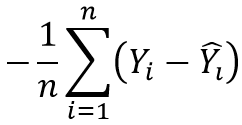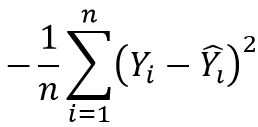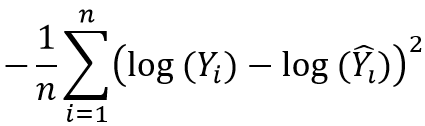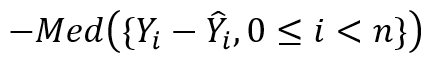## 9 Automated Machine Learning

Use the automated algorithm selection, feature selection, and hyperparameter tuning of Automated Machine Learning to accelerate the machine learning modeling process.

Automated Machine Learning in OML4Py is described in the following topics:

### 9.1 About Automated Machine Learning

Automated Machine Learning (AutoML) provides built-in data science expertise about data analytics and modeling that you can employ to build machine learning models.

Any modeling problem for a specified data set and prediction task involves a sequence of data cleansing and preprocessing, algorithm selection, and model tuning tasks. Each of these steps require data science expertise to help guide the process to an efficient final model. Automated Machine Learning (AutoML) automates this process with its built-in data science expertise.

OML4Py has the following AutoML capabilities:

• Automated algorithm selection that selects the appropriate algorithm from the supported machine learning algorithms
• Automated feature selection that reduces the size of the original feature set to speed up model training and tuning, while possibly also increasing model quality
• Automated tuning of model hyperparameters, which selects the model with the highest score metric from among several metrics as selected by the user

AutoML performs those common modeling tasks automatically, with less effort and potentially better results. It also leverages in-database algorithm parallel processing and scalability to minimize runtime and produce high-quality results.

Note:

As the `fit` method of the machine learning classes does, the AutoML functions `reduce`, `select`, and `tune` provide a `case_id` parameter that you can use to achieve repeatable data sampling and data shuffling during model building.

The AutoML functionality is also available in a no-code user interface alongside OML Notebooks on Oracle Autonomous Database. For more information, see Oracle Machine Learning AutoML User Interface .

Automated Machine Learning Classes and Algorithms

The Automated Machine Learning classes are the following.

Class Description
`oml.automl.AlgorithmSelection`

Using only the characteristics of the data set and the task, automatically selects the best algorithms from the set of supported Oracle Machine Learning algorithms.

Supports classification and regression functions.

`oml.automl.FeatureSelection`

Uses meta-learning to quickly identify the most relevant feature subsets given a training data set and an Oracle Machine Learning algorithm.

Supports classification and regression functions.

`oml.automl.ModelTuning`

Uses a highly parallel, asynchronous gradient-based hyperparameter optimization algorithm to tune the algorithm hyperparameters.

Supports classification and regression functions.

`oml.automl.ModelSelection`

Selects the best Oracle Machine Learning algorithm and then tunes that algorithm.

Supports classification and regression functions.

The Oracle Machine Learning algorithms supported by AutoML are the following:

Table 9-1 Machine Learning Algorithms Supported by AutoML

Algorithm Abbreviation Algorithm Name
dt Decision Tree
glm Generalized Linear Model
glm_ridge Generalized Linear Model with ridge regression
nb Naive Bayes
nn Neural Network
rf Random Forest
svm_gaussian Support Vector Machine with Gaussian kernel
svm_linear Support Vector Machine with linear kernel

Classification and Regression Metrics

The following tables list the scoring metrics supported by AutoML.

Table 9-2 Binary and Multiclass Classification Metrics

Metric Description, Scikit-learn Equivalent, and Formula
`accuracy`

Calculates the rate of correct classification of the target.

``sklearn.metrics.accuracy_score(y_true, y_pred, normalize=True, sample_weight=None)``

Formula: `(tp + tn)/samples`

`f1_macro`

Calculates the f-score or f-measure, which is a weighted average of the precision and recall. The f1_macro takes the unweighted average of per-class scores.

``sklearn.metrics.f1_score(y_true, y_pred, labels=None, pos_label=1, average=’macro’, sample_weight=None)``

Formula: ```2 * (precision * recall) / (precision + recall)```

`f1_micro`

Calculates the f-score or f-measure with micro-averaging in which true positives, false positives, and false negatives are counted globally.

``sklearn.metrics.f1_score(y_true, y_pred, labels=None, pos_label=1, average=’micro’, sample_weight=None)``

Formula: ```2 * (precision * recall) / (precision + recall)```

`f1_weighted`

Calculates the f-score or f-measure with weighted averaging of per-class scores based on support (the fraction of true samples per class). Accounts for imbalanced classes.

``sklearn.metrics.f1_score(y_true, y_pred, labels=None, pos_label=1, average=’weighted’, sample_weight=None)``

Formula: ```2 * (precision * recall) / (precision + recall)```

`precision_macro`

Calculates the ability of the classifier to not label a sample incorrectly. The precision_macro takes the unweighted average of per-class scores.

``sklearn.metrics.precision_score(y_true, y_pred, labels=None, pos_label=1, average=’macro’, sample_weight=None)``

Formula: `tp / (tp + fp)`

`precision_micro`

Calculates the ability of the classifier to not label a sample incorrectly. Uses micro-averaging in which true positives, false positives, and false negatives are counted globally.

``sklearn.metrics.precision_score(y_true, y_pred, labels=None, pos_label=1, average=’micro’, sample_weight=None)``

Formula: `tp / (tp + fp)`

`precision_weighted`

Calculates the ability of the classifier to not label a sample incorrectly. Uses weighted averaging of per-class scores based on support (the fraction of true samples per class). Accounts for imbalanced classes.

``sklearn.metrics.precision_score(y_true, y_pred, labels=None, pos_label=1, average=’weighted’, sample_weight=None)``

Formula: `tp / (tp + fp)`

`recall_macro`

Calculates the ability of the classifier to correctly label each class. The recall_macro takes the unweighted average of per-class scores.

``sklearn.metrics.recall_score(y_true, y_pred, labels=None, pos_label=1, average=’macro’, sample_weight=None)``

Formula: `tp / (tp + fn)`

`recall_micro`

Calculates the ability of the classifier to correctly label each class with micro-averaging in which the true positives, false positives, and false negatives are counted globally.

``sklearn.metrics.recall_score(y_true, y_pred, labels=None, pos_label=1, average=’micro’, sample_weight=None)``

Formula: `tp / (tp + fn)`

`recall_weighted`

Calculates the ability of the classifier to correctly label each class with weighted averaging of per-class scores based on support (the fraction of true samples per class). Accounts for imbalanced classes.

``sklearn.metrics.recall_score(y_true, y_pred, labels=None, pos_label=1, average=’weighted’, sample_weight=None)``

Formula: `tp / (tp + fn)`

Table 9-3 Binary Classification Metrics Only

Metric Description, Scikit-learn Equivalent, and Formula
`f1`

Calculates the f-score or f-measure, which is a weighted average of the precision and recall. This metric by default requires a positive target to be encoded as 1 to function as expected.

``sklearn.metrics.f1_score(y_true, y_pred, labels=None, pos_label=1, average=’binary’, sample_weight=None)``

Formula: ```2 * (precision * recall) / (precision + recall)```

`precision`

Calculates the ability of the classifier to not label a sample positive (1) that is actually negative (0).

``sklearn.metrics.precision_score(y_true, y_pred, labels=None, pos_label=1, average=’binary’, sample_weight=None)``

Formula: `tp / (tp + fp)`

`recall`

Calculates the ability of the classifier to label all positive (1) samples correctly.

``sklearn.metrics.recall_score(y_true, y_pred, labels=None, pos_label=1, average=’binary’, sample_weight=None)``

Formula: `tp / (tp + fn)`

`roc_auc`

Calculates the Area Under the Receiver Operating Characteristic Curve (roc_auc) from prediction scores.

``sklearn.metrics.accuracy_score(y_true, y_pred, normalize=True, sample_weight=None)``

Table 9-4 Regression Metrics

Metric Description, Scikit-learn Equivalent, and Formula
`r2`

Calculates the coefficient of determination (R squared).

``sklearn.metrics.r2_score(y_true, y_pred, sample_weight=None, multioutput=’uniform_average’)``

`neg_mean_absolute_error`

Calculates the mean of the absolute difference of predicted and true targets (MAE).

``sklearn.metrics.mean_absolute_error(y_true, y_pred, sample_weight=None, multioutput=’uniform_average’)``

Formula:Description of the illustration negmeanabserr.png
`neg_mean_squared_error`

Calculates the mean of the squared difference of predicted and true targets.

``-1.0 * sklearn.metrics.mean_squared_error(y_true, y_pred, sample_weight=None, multioutput=’uniform_average’)``

Formula:Description of the illustration negmeansqerr.png
`neg_mean_squared_log_error`

Calculates the mean of the difference in the natural log of predicted and true targets.

``sklearn.metrics.mean_squared_log_error(y_true, y_pred, sample_weight=None, multioutput=’uniform_average’)``

Formula:Description of the illustration negmeansqlogerr.png
`neg_median_absolute_error`

Calculates the median of the absolute difference between predicted and true targets.

``sklearn.metrics.median_absolute_error(y_true, y_pred)``

Formula:Description of the illustration negmedianabserr.png

### 9.2 Algorithm Selection

The `oml.automl.AlgorithmSelection` class uses the characteristics of the data set and the task to rank algorithms from the set of supported Oracle Machine Learning algorithms.

Selecting the best Oracle Machine Learning algorithm for a data set and a prediction task is non-trivial. No single algorithm works best for all modeling problems. The `oml.automl.AlgorithmSelection` class ranks the candidate algorithms according to how likely each is to produce a quality model. This is achieved by using Oracle advanced meta-learning intelligence learned from a repertoire of data sets with the goal of avoiding exhaustive searches, thereby reducing overall compute time and costs.

The `oml.automl.AlgorithmSelection` class supports classification and regression algorithms. To use the class, you specify a data set and the number of algorithms you want to evaluate.

The `select` method of the class returns a sorted list of the top algorithms and their predicted rank (from best to worst).

For information on the parameters and methods of the class, invoke `help(oml.automl.AlgorithmSelection)` or see Oracle Machine Learning for Python API Reference.

Example 9-1 Using the `oml.automl.AlgorithmSelection` Class

This example creates an `oml.automl.AlgorithmSelection` object and then displays the algorithm rankings with their corresponding score metric. You may select the top entry or choose a different model depending on the needs of your particular business problem.

``````import oml
from oml import automl
import pandas as pd
from sklearn import datasets

# Load the breast cancer data set.
bc_data = bc.data.astype(float)
X = pd.DataFrame(bc_data, columns = bc.feature_names)
y = pd.DataFrame(bc.target, columns = ['TARGET'])

# Create the database table BreastCancer.
oml_df = oml.create(pd.concat([X, y], axis=1),
table = 'BreastCancer')

# Split the data set into training and test data.
train, test = oml_df.split(ratio=(0.8, 0.2), seed = 1234)
X, y = train.drop('TARGET'), train['TARGET']
X_test, y_test = test.drop('TARGET'), test['TARGET']

# Create an automated algorithm selection object with f1_macro as
# the score_metric argument.
asel = automl.AlgorithmSelection(mining_function='classification',
score_metric='f1_macro', parallel=4)

# Run algorithm selection to get the top k predicted algorithms and
# their ranking without tuning.
algo_ranking = asel.select(X, y, k=3)

# Show the selected and tuned model.
[(m, "{:.2f}".format(s)) for m,s in algo_ranking]

# Drop the database table.
oml.drop('BreastCancer')``````

Listing for This Example

### 9.3 Feature Selection

The `oml.automl.FeatureSelection` class identifies the most relevant feature subsets for a training data set and an Oracle Machine Learning algorithm.

In a data analytics application, feature selection is a critical data preprocessing step that has a high impact on both runtime and model performance. The `oml.automl.FeatureSelection` class automatically selects the most relevant features for a data set and model. It internally uses several feature-ranking algorithms to identify the best feature subset that reduces model training time without compromising model performance. Oracle advanced meta-learning techniques quickly prune the search space of this feature selection optimization.

The `oml.automl.FeatureSelection` class supports classification and regression algorithms. To use the `oml.automl.FeatureSelection` class, you specify a data set and the Oracle Machine Learning algorithm on which to perform the feature reduction.

For information on the parameters and methods of the class, invoke `help(oml.automl.FeatureSelection)` or see Oracle Machine Learning for Python API Reference.

Example 9-2 Using the `oml.automl.FeatureSelection` Class

This example uses the `oml.automl.FeatureSelection` class. The example builds a model on the full data set and computes predictive accuracy. It performs automated feature selection, filters the columns according to the determined set, and rebuilds the model. It then recomputes predictive accuracy.

``````import oml
from oml import automl
import pandas as pd
import numpy as np
from sklearn import datasets

# Load the digits data set into the database.
X = pd.DataFrame(digits.data,
columns = ['pixel{}'.format(i) for i
in range(digits.data.shape)])
y = pd.DataFrame(digits.target, columns = ['digit'])
oml_df = oml.create(pd.concat([X, y], axis=1), table = 'DIGITS')

# Split the data set into train and test.
train, test = oml_df.split(ratio=(0.8, 0.2),
seed = 1234, strata_cols='digit')
X_train, y_train = train.drop('digit'), train['digit']
X_test, y_test = test.drop('digit'), test['digit']

# Default model performance before feature selection.
mod = oml.svm(mining_function='classification').fit(X_train,
y_train)
"{:.2}".format(mod.score(X_test, y_test))

# Create an automated feature selection object with accuracy
# as the score_metric.
fs = automl.FeatureSelection(mining_function='classification',
score_metric='accuracy', parallel=4)

# Get the reduced feature subset on the train data set.
subset = fs.reduce('svm_linear', X_train, y_train)
"{} features reduced to {}".format(len(X_train.columns),
len(subset))

# Use the subset to select the features and create a model on the
# new reduced data set.
X_new  = X_train[:,subset]
X_test_new = X_test[:,subset]
mod = oml.svm(mining_function='classification').fit(X_new, y_train)
"{:.2} with {:.1f}x feature reduction".format(
mod.score(X_test_new, y_test),
len(X_train.columns)/len(X_new.columns))

# Drop the DIGITS table.
oml.drop('DIGITS')

# For reproducible results, add a case_id column with unique row
# identifiers.
row_id = pd.DataFrame(np.arange(digits.data.shape),
columns = ['CASE_ID'])
oml_df_cid = oml.create(pd.concat([row_id, X, y], axis=1),
table = 'DIGITS_CID')

train, test = oml_df_cid.split(ratio=(0.8, 0.2), seed = 1234,
hash_cols='CASE_ID',
strata_cols='digit')
X_train, y_train = train.drop('digit'), train['digit']
X_test, y_test = test.drop('digit'), test['digit']

# Provide the case_id column name to the feature selection
# reduce function.
subset = fs.reduce('svm_linear', X_train,
y_train, case_id='CASE_ID')
"{} features reduced to {} with case_id".format(
len(X_train.columns)-1,
len(subset))

# Drop the tables created in the example.
oml.drop('DIGITS')
oml.drop('DIGITS_CID')``````

Listing for This Example

### 9.4 Model Tuning

The `oml.automl.ModelTuning` class tunes the hyperparameters for the specified classification or regression algorithm and training data.

Model tuning is a laborious machine learning task that relies heavily on data scientist expertise. With limited user input, the `oml.automl.ModelTuning` class automates this process using a highly-parallel, asynchronous gradient-based hyperparameter optimization algorithm to tune the hyperparameters of an Oracle Machine Learning algorithm.

The `oml.automl.ModelTuning` class supports classification and regression algorithms. To use the `oml.automl.ModelTuning` class, you specify a data set and an algorithm to obtain a tuned model and its corresponding hyperparameters. An advanced user can provide a customized hyperparameter search space and a non-default scoring metric to this black box optimizer.

For a partitioned model, if you pass in the column to partition on in the `param_space` argument of the `tune` method, `oml.automl.ModelTuning` tunes the partitioned model’s hyperparameters.

For information on the parameters and methods of the class, invoke `help(oml.automl.ModelTuning)` or see Oracle Machine Learning for Python API Reference.

Example 9-3 Using the `oml.automl.ModelTuning` Class

This example creates an `oml.automl.ModelTuning` object.

``````import oml
from oml import automl
import pandas as pd
from sklearn import datasets

# Load the breast cancer data set.
bc_data = bc.data.astype(float)
X = pd.DataFrame(bc_data, columns = bc.feature_names)
y = pd.DataFrame(bc.target, columns = ['TARGET'])

# Create the database table BreastCancer.
oml_df = oml.create(pd.concat([X, y], axis=1),
table = 'BreastCancer')

# Split the data set into training and test data.
train, test = oml_df.split(ratio=(0.8, 0.2), seed = 1234)
X, y = train.drop('TARGET'), train['TARGET']
X_test, y_test = test.drop('TARGET'), test['TARGET']

# Start an automated model tuning run with a Decision Tree model.
at = automl.ModelTuning(mining_function='classification',
parallel=4)
results = at.tune('dt', X, y, score_metric='accuracy')

# Show the tuned model details.
tuned_model = results['best_model']
tuned_model

# Show the best tuned model train score and the
# corresponding hyperparameters.
score, params = results['all_evals']
"{:.2}".format(score), ["{}:{}".format(k, params[k])
for k in sorted(params)]

# Use the tuned model to get the score on the test set.
"{:.2}".format(tuned_model.score(X_test, y_test))

# An example invocation of model tuning with user-defined
# search ranges for selected hyperparameters on a new tuning
# metric (f1_macro).
search_space = {
'RFOR_SAMPLING_RATIO': {'type': 'continuous',
'range': [0.01, 0.5]},
'RFOR_NUM_TREES': {'type': 'discrete',
'range': [50, 100]},
'TREE_IMPURITY_METRIC': {'type': 'categorical',
'range': ['TREE_IMPURITY_ENTROPY',
'TREE_IMPURITY_GINI']},}
results = at.tune('rf', X, y, score_metric='f1_macro',
param_space=search_space)
score, params = results['all_evals']
("{:.2}".format(score), ["{}:{}".format(k, params[k])
for k in sorted(params)])

# Some hyperparameter search ranges need to be defined based on the
# training data set sizes (for example, the number of samples and
# features). You can use placeholders specific to the data set,
# such as \$nr_features and \$nr_samples, as the search ranges.
search_space = {'RFOR_MTRY': {'type': 'discrete',
'range': [1, '\$nr_features/2']}}
results = at.tune('rf', X, y,
score_metric='f1_macro', param_space=search_space)
score, params = results['all_evals']
("{:.2}".format(score), ["{}:{}".format(k, params[k])
for k in sorted(params)])

# Drop the database table.
oml.drop('BreastCancer')``````

Listing for This Example

### 9.5 Model Selection

The `oml.automl.ModelSelection` class automatically selects an Oracle Machine Learning algorithm according to the selected score metric and then tunes that algorithm.

The `oml.automl.ModelSelection` class supports classification and regression algorithms. To use the `oml.automl.ModelSelection` class, you specify a data set and the number of algorithms you want to tune.

The `select` method of the class returns the best model out of the models considered.

For information on the parameters and methods of the class, invoke `help(oml.automl.ModelSelection)` or see Oracle Machine Learning for Python API Reference.

Example 9-4 Using the `oml.automl.ModelSelection` Class

This example creates an `oml.automl.ModelSelection` object and then uses the object to select and tune the best model.

``````import oml
from oml import automl
import pandas as pd
from sklearn import datasets

# Load the breast cancer data set.
bc_data = bc.data.astype(float)
X = pd.DataFrame(bc_data, columns = bc.feature_names)
y = pd.DataFrame(bc.target, columns = ['TARGET'])

# Create the database table BreastCancer.
oml_df = oml.create(pd.concat([X, y], axis=1),
table = 'BreastCancer')

# Split the data set into training and test data.
train, test = oml_df.split(ratio=(0.8, 0.2), seed = 1234)
X, y = train.drop('TARGET'), train['TARGET']
X_test, y_test = test.drop('TARGET'), test['TARGET']

# Create an automated model selection object with f1_macro as the
# score_metric argument.
ms = automl.ModelSelection(mining_function='classification',
score_metric='f1_macro', parallel=4)

# Run model selection to get the top (k=1) predicted algorithm
# (defaults to the tuned model).
select_model = ms.select(X, y, k=1)

# Show the selected and tuned model.
select_model

# Score on the selected and tuned model.
"{:.2}".format(select_model.score(X_test, y_test))

# Drop the database table.
oml.drop('BreastCancer')``````

Listing for This Example